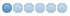# I do not find "base period" or "motor steps per rev" in my .ini File

21 Jun 2021 22:13 #212603 by BigJohnT
That is just for your information, I'm not sure how it's calculated but the code is there.

github.com/LinuxCNC/linuxcnc/blob/master...nf/build_INI.py#L205

github.com/LinuxCNC/linuxcnc/blob/master...nf/build_INI.py#L388

JT

• Roman Simon
• Offline
• New Member
•22 Jun 2021 07:07 #212648
thank you, I think figure it out.

The written base period in *.ini is in my case not calculated on max jitter.
ideal_period() with 4 axes takes the maximum value of hz() for x or y (in my case 4800) and
because I have doublestep() with my values, a high number is calculated (1000000000 / 4800 = 208333)
and limited to 100000. 100000 is not smaller than the max jitter calculation (with minperiod())
so base period is always 100000.

Here are the code snippets, main part is at the end in ideal_period()
```// buid_INI.py

base_period = self.d.ideal_period()

// stepconf.py

self.steptime = 5000 // *.stepconf
self.stepspace = 5000 // *.stepconf

def hz(self, axname):
steprev = getattr(self, axname+"steprev") // for x 200.0
microstep = getattr(self, axname+"microstep") // for x 2
pulleynum = getattr(self, axname+"pulleynum") // for x 1.0
pulleyden = getattr(self, axname+"pulleyden") // for x 1
maxvel = getattr(self, axname+"maxvel") // for x 12.0
if self.units or axname == 'a': leadscrew = 1./leadscrew // for a 360.0
pps = leadscrew * steprev * microstep * (pulleynum/pulleyden) * maxvel
return abs(pps)

def doublestep(self, steptime=None):
if steptime is None: steptime = self.steptime
return steptime <= 5000

def minperiod(self, steptime=None, stepspace=None, latency=None):
if steptime is None: steptime = self.steptime
if stepspace is None: stepspace = self.stepspace
if latency is None: latency = self.latency
if self.doublestep(steptime):
return max(latency + steptime + stepspace + 5000, 4*steptime)
else:
return latency + max(steptime, stepspace)

def ideal_period(self):
xhz = self.hz('x')
yhz = self.hz('y')
zhz = self.hz('z')
uhz = self.hz('u')
vhz = self.hz('v')
ahz = self.hz('a')
if self.axes == 1:
pps = max(xhz, yhz, zhz, ahz)
elif self.axes == 0:
pps = max(xhz, yhz, zhz)
elif self.axes == 2:
pps = max(xhz, zhz)
elif self.axes == 3:
pps = max(xhz, yhz, uhz, vhz)
elif self.axes == 4:  // YES
pps = max(xhz, yhz)
else:
print('error in ideal period calculation - number of axes unrecognized')
return

// pss = 4800

if self.doublestep():
base_period = 1e9 / pps   // 1000000000 / 4800 = 208333
else:
base_period = .5e9 / pps
if base_period > 100000: base_period = 100000
if base_period < self.minperiod(): base_period = self.minperiod()
return int(base_period)```

I would like to check if I have missing steps. When I fastly move X
20 Minutes from left to right, can I simply check it with an indicator, if
startpoint and endpoint is different?

More axis means more work, so base period must be higher?
When a calculation do not fit in a base period, does it come delayed
or is it skipped? I think this also a very important point.

22 Jun 2021 11:43 #212667 by BigJohnT
The following user(s) said Thank You: Roman Simon

22 Jun 2021 11:53 #212669
When checking an axis be sure to take up any backlash before hand.
The following user(s) said Thank You: Roman Simon

22 Jun 2021 11:57 #212671 by andypugh
I think that the point is that you need a base thread frequency high enough to handle the maximum required step rate, but there isn't that much to be gained from having it much higher, and the disadvantage is that there is quite a waste of CPU time.

So Stepconf uses 100,000 if 100,000 is short enough to reach the required axis speeds.

If you were to increase the axis speeds in stepconf then I think that you would eventually see the base period reduce.

That said, there is _something_ to be said for a shorter than necessary base period. Consider the case where you are stepping every base thread period at 100,000ns, ie 10kHz. The next speed down without dithering is 5khz, ie stepping every second period. This is partly why Mesa and Pico cards can appear smoother, they have a base clock in the MHz range, and so can make fractional changes.. 10kHz - 10.001kHz...10.002kHz etc.
The following user(s) said Thank You: Roman Simon

•